## Abstract

We present a general formulation for incompressible fluid flow analysis using the finite element method (FEM) and a lagrangian description. The necessary stabilization for dealing with the incompressibility condition is introduced via the so called finite calculus (FIC) method. Both a quasi-implicit algorithm and a fractional step scheme are described. Examples of application of the lagrangian flow description are presented.

Keywords: Lagrangian formulation, incompressible fluid flow, finite calculus, finite element method.

## 1 INTRODUCTION

The development of efficient and robust numerical methods such as the finite element, finite volume and finite difference methods for analysis of incompressible flows has been a subject of intensive research in last decades [1,2]. Much effort has been spent in developing the so called stabilized numerical methods overcoming the two main sources of instability in incompressible flow analysis, namely those originated by the high values of the convective terms and those induced by the difficulty in satisfying the incompressibility conditions.

The problems originated by the convective terms dissapear if a lagrangian description is used. In the lagrangian formulation the motion of each individual flow particle is followed as in solid mechanics problems. The difficulty is tranferred now to the problem if adequately moving the mesh nodes. Indeed for large mesh motions remeshing is necessary after flow time steps.

A popular way to overcome the problems with the incompressibility constraint is by introducing a pseudo-compressibility in the flow and using implicit and explicit algorithms developed for this kind of problems such as pressure segregation schemes , artificial compressibility methods [4-6] and preconditioning techniques . Other FEM schemes with good stabilization properties for the incompressibility terms are based in Petrov-Galerkin (PG) techniques. The background of PG methods are the non-centred (upwind) schemes for computing the first derivatives of the convective operator in FD and FV methods [2,8]. More recently a general class of Galerkin FEM has been developed where the standard Galerkin variational form is extended with adequate residual-based terms in order to achieve a stabilized numerical scheme. Among the many methods of this kind we can name the Streamline Upwind Petrov Galerkin (SUPG) method [1,9-18] the Galerkin Least Square (GLS) method [19,20], the Taylor-Galerkin method , the Characteristic Galerkin method [22-24] and its variant the Characteristic Based Split (CBS) method [25,26] the pressure gradient operator technique  and the Subgrid Scale (SS) method [28-30]. A good review of these methods can be found in .

In this paper a stabilized finite element formulation for incompressible lagrangian flows is derived in a different manner. The starting point are the modified governing differential equations of the fluid flow problem formulated via a finite calculus (FIC) approach . The FIC method is based in invoking the balance of fluxes in a fluid domain of finite size. This introduces naturally additional terms in the classical differential equations of infinitesimal fluid mechanics which are a function of the balance domain dimensions. The new terms in the modified governing equations provide the necessary stabilization to the discrete equations obtained via the standard Galerkin finite element method [33-39].

The layout of the chapter is the following. In the next section, the main concepts of the FIC approach are introduced via a simple 1D convection-diffusion model problem. Then the basic FIC equations for incompressible lagrangian flow problems are presented. The finite element discretization is introduced and the resulting matrix formulation is detailed. Both monolithic and fractional step schemes for the transient solution are presented.

The lagrangian description has many advantages for tracking the displacement of fluid particles in flows where large motions of the fluid surface occur such in the case of breaking waves, splashing of water, filling of moulds, etc. As above mentioned, the positive feature of the lagrangian formulation is that the convective terms dissapear in the governing equations of the fluid flow; in return the updating of the mesh at almost every time step is now a necessity and an efficient algorithm for generation of a finite element mesh must be used.

The examples show the efficiency of the lagrangian formulation to solve fluid flow problems involving contact with moving solids, surface waves around ships and large motions of the free surface, among others.

## 2 THE FINITE CALCULUS METHOD

We will consider a convection-diffusion problem in a 1D domain ${\textstyle \Omega }$ of length ${\textstyle L}$. The equation of balance of fluxes in a subdomain of size ${\textstyle d}$ belonging to ${\textstyle \Omega }$ (Figure 1) is written as

 $q_{A}-q_{B}=0$
(1)

where ${\textstyle q_{A}}$ and ${\textstyle q_{B}}$ are the incoming and outgoing fluxes at points ${\textstyle A}$ and ${\textstyle B}$, respectively. The flux ${\textstyle q}$ includes both convective and diffusive terms; i.e. ${\textstyle q=u\phi -k{d\phi \over dx}}$, where ${\textstyle \phi }$ is the transported variable, ${\textstyle v}$ is the velocity and ${\textstyle k}$ is the diffusitivity of the material.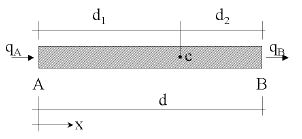Figure 1: Equilibrium of fluxes in a balance domain of finite size

Let us express now the fluxes ${\textstyle q_{A}}$ and ${\textstyle q_{B}}$ in terms of the flux at an arbitrary point ${\textstyle C}$ within the balance domain (Figure 1). Expanding ${\textstyle q_{A}}$ and ${\textstyle q_{B}}$ in Taylor series around point ${\textstyle C}$ up to second order terms gives

 $q_{A}=q_{C}-d_{1}{\frac {dq}{dx}}\vert _{C}+{\frac {d_{1}^{2}}{2}}{\frac {d^{2}q}{dx^{2}}}\vert _{C}+O(d_{1}^{3})\quad ,\quad q_{B}=q_{C}+d_{2}{\frac {dq}{dx}}\vert _{C}+{\frac {d_{2}^{2}}{2}}{\frac {d^{2}q}{dx^{2}}}\vert _{C}+O(d_{2}^{3})$
(2)

Substituting eq.(2) into eq.(1) gives after simplification

 ${\frac {dq}{dx}}-{\underline {{\frac {h}{2}}{\frac {d^{2}q}{dx^{2}}}}}=0$
(3)

where ${\textstyle h=d_{1}-d_{2}}$ and all derivatives are computed at point ${\textstyle C}$.

Standard calculus theory assumes that the domain ${\textstyle d}$ is of infinitesimal size and the resulting balance equation is simply ${\textstyle {dq \over dx}=0}$. We will relax this assumption and allow the balance domain to have a finite size. The new balance equation (3) incorporates now the underlined term which introduces the characteristic length ${\textstyle h}$. Obviously, accounting for higher order terms in eq.(2) would lead to new terms in eq.(3) involving higher powers of ${\textstyle h}$.

Distance ${\textstyle h}$ in eq.(3) can be interpreted as a free parameter depending, of course, on the location of point ${\textstyle C}$ (note that ${\textstyle -d\leq h\leq d}$). However, the fact that eq.(3) is the exact balance equation (up to second order terms) for any 1D domain of finite size and that the position of point ${\textstyle C}$ is arbitrary, can be used to derive numerical schemes with enhanced properties simply by computing the characteristic length parameter from an adequate “optimality” rule.

Equation (3) can be extended to account for source effects. The full stabilized equation can be then written in compact form as

 $r-{\underline {{\frac {h}{2}}{\frac {dr}{dx}}}}=0$
(4)

with

 $r=-u{\frac {d\phi }{dx}}+{\frac {d}{dx}}\left(k{\frac {d\phi }{dx}}\right)+Q$
(5)

where ${\textstyle Q}$ is the external source. For consistency a “finite” form of the Neumann boundary condition should be used. This can be readily obtained by invoking balance of fluxes in a domain of finite size next to the boundary ${\textstyle \Gamma _{q}}$ where the external (diffusive) flux is prescribed to a value ${\textstyle q_{p}}$. The modified Neumann boundary condition can be written as 

 $k{\frac {d\phi }{dx}}+q_{p}-{\underline {{\frac {h}{2}}r}}=0\quad {\hbox{at }}\,\Gamma _{q}$
(6)

The definition of the problem is completed with the standard Dirichlet condition prescribing the value of ${\textstyle \phi }$ at the boundary ${\textstyle \Gamma _{\phi }}$.

The underlined terms in Eqs.(5) and (7) introduce the necessary stabilization in the discrete solution of the problem using whatever numerical scheme. For details see [32-41].

The starting point in the next section are the FIC equation for a viscous incompressible fluid written in a lagrangian frame of reference.

## 3 FIC EQUATIONS FOR VISCOUS INCOMPRESSIBLE FLOW USING A LAGRANGIAN DESCRIPTION

The FIC governing equations for a viscous incompressible fluid can be written in a lagrangian frame as

Momentum

 $r_{m_{i}}-{\underline {{1 \over 2}h_{j}{\partial r_{m_{i}} \over \partial x_{j}}}}=0$
(7)

Mass balance

 $r_{d}-{\underline {{1 \over 2}h_{j}{\partial r_{d} \over \partial x_{j}}}}=0$
(8)

where

 $r_{m_{i}}=\rho {\partial u_{i} \over \partial t}+{\partial p \over \partial x_{i}}-{\partial s_{ij} \over \partial x_{j}}-b_{i}$
(9)

 $r_{d}={\partial u_{i} \over \partial x_{i}}\qquad i,j=1,n_{d}$
(10)

Above ${\textstyle u_{i}}$ is the velocity along the ith global axis, ${\textstyle \rho }$ is the (constant) density of the fluid, ${\textstyle p}$ is the absolute pressure (defined positive in compression), ${\textstyle b_{i}}$ are the body forces and ${\textstyle s_{ij}}$ are the viscous deviatoric stresses related to the viscosity ${\textstyle \mu }$ by the standard expression

 $s_{ij}=2\mu \left({\dot {\varepsilon }}_{ij}-\delta _{ij}{1 \over 3}{\partial u_{k} \over \partial x_{k}}\right)$
(11)

where ${\textstyle \delta _{ij}}$ is the Kronecker delta and the strain rates ${\textstyle {\dot {\varepsilon }}_{ij}}$ are

 ${\dot {\varepsilon }}_{ij}={1 \over 2}\left({\partial u_{i} \over \partial x_{j}}+{\partial u_{j} \over \partial x_{i}}\right)$
(12)

The FIC boundary conditions are

 $n_{j}\sigma _{ij}-t_{i}+{\underline {{1 \over 2}h_{j}n_{j}r_{m_{i}}}}=0\quad {\hbox{on }}\Gamma _{t}$
(13)

 $u_{j}-u_{j}^{p}=0\quad {\hbox{on }}\Gamma _{u}$
(14)

and the initial condition is ${\textstyle u_{j}=u_{j}^{0}}$ for ${\textstyle t=t_{0}}$.

In Eqs.(13) and (14) ${\textstyle t_{i}}$ and ${\textstyle u_{j}^{p}}$ are surface tractions and prescribed displacements on the boundaries ${\textstyle \Gamma _{t}}$ and ${\textstyle \Gamma _{u}}$, respectively, ${\textstyle n_{j}}$ are the components of the unit normal vector to the boundary and ${\textstyle \sigma _{ij}}$ are the total stresses given by ${\textstyle \sigma _{ij}=s_{ij}-\delta _{ij}p}$. The sign in front the stabilization term in Eq.(13) is positive due to the definition of ${\textstyle r_{m_{i}}}$ in Eq.(9).

The ${\textstyle h_{i}'s}$ in above equations are characteristic lengths of the domain where balance of momentum and mass is enforced. In Eq.(13) these lengths define the domain where equilibrium of boundary tractions is established .

Eqs.(7)–(14) are the starting point for deriving stabilized finite element methods for solving the incompressible Navier-Stokes equations in a lagrangian frame of reference using equal order interpolation for the velocity and pressure variables [37-39]. Application of the FIC formulations to meshless analysis of fluid flow problems using the finite point method can be found in [40,41].

### 3.1 Stabilized integral forms

From Eq.(7) it can be obtained (taking into account Eqs.(9) and (11))

 ${\partial r_{d} \over \partial x_{i}}=-{1 \over a_{i}}\left[{\bar {r}}_{m_{i}}-{h_{j} \over 2}{\partial r_{m_{i}} \over \partial x_{j}}\right]-{\rho u_{i}h_{k} \over 2a_{i}}{\partial r_{d} \over \partial x_{k}}\quad i,j=1,n_{d}\quad ,\quad k\not =i$
(15)

where

 $a_{i}={2\mu \over 3}$
(16.a)

and

 ${\bar {r}}_{m_{i}}=\rho {\partial u_{i} \over \partial t}+{\partial p \over \partial x_{i}}-{\partial \over \partial x_{j}}(2\mu {\dot {\varepsilon }}_{ij})-b_{i}$
(16.b)

Substituting Eq.(15) into Eq.(8) and retaining the terms involving the derivatives of ${\textstyle r_{m_{i}}}$ with respect to ${\textstyle x_{i}}$ only, leads to the following expression for the stabilized mass balance equation

 $\displaystyle {r_{d}-\sum \limits _{i=1}^{n_{d}}\tau _{i}{\partial r_{m_{i}} \over \partial x_{i}}=0}$
(17)

with

 $\tau _{i}={3h_{i}^{2} \over 8\mu }$
(18)

The ${\textstyle \tau _{i}}$'s in Eq.(17) are termed in the stabilization literature intrinsic time parameters. Similar values for ${\textstyle \tau _{i}}$ (usually ${\textstyle \tau _{i}=\tau }$ is taken) are used in other works from ad-hoc extensions of the 1D advective-diffusive problem [12-31]. It is remarkable that the intrinsic time parameters have been deduced here from the general FIC formulation and this shows the possibilities of the method.

The weighted residual form of the momentum and mass balance equations (Eqs.(7) and (17)) is written as

Momentum

 $\int _{\Omega }\delta u_{i}\left[r_{m_{i}}-{h_{j} \over 2}{\partial r_{m_{i}} \over \partial x_{j}}\right]+\int _{\Gamma _{t}}\delta u_{i}(\sigma _{ij}n_{j}-t_{i}+{h_{j} \over 2}n_{j}r_{m_{i}})d\Gamma =0$
(19)

Mass balance

 $\int _{\Omega }q\left[r_{d}-\sum \limits _{i=1}^{n_{d}}\tau _{i}{\partial r_{m_{i}} \over \partial x_{i}}\right]d\Omega =0$
(20)

where ${\textstyle \delta u_{i}}$ and ${\textstyle q}$ are arbitrary weighting functions representing virtual velocity and virtual pressure fields. Integration by parts of the ${\textstyle r_{m_{i}}}$ terms leads to

 $\int _{\Omega }\delta u_{i}r_{m_{i}}+\int _{\Gamma _{t}}\delta u_{i}(\sigma _{ij}n_{j}-t_{i})d\Gamma +\sum \limits _{e}\int _{\Omega ^{e}}{h_{j} \over 2}{\partial \delta u_{i} \over \partial x_{j}}r_{m_{i}}d\Omega =0$
(21.a)

 $\int _{\Omega }qr_{d}d\Omega +\int _{\Omega }\left[\sum \limits _{i=1}^{n_{d}}\tau _{i}{\partial q \over \partial x_{i}}r_{m_{i}}\right]d\Omega -\int _{\Gamma }\left[\sum \limits _{i=1}^{n_{d}}q\tau _{i}n_{i}r_{m_{i}}\right]d\Gamma =0$
(21.b)

The third integral in Eq.(21.a) is expressed as a sum of the element contributions to allow for discontinuities in the derivatives of ${\textstyle r_{m_{i}}}$ along the element interfaces.

Also in Eq.(21.a) we will neglect hereonwards the third integral by assuming that ${\textstyle r_{m_{i}}}$ is negligible on the boundaries. The deviatoric stresses and the pressure terms in the first integral of Eq.(21.a) are integrated by parts in the usual manner. The resulting equations are

Momentum

 $\int _{\Omega }\left[\delta u_{i}\rho {\partial u_{i} \over \partial t}+\delta {\dot {\varepsilon }}_{ij}(s_{ij}-\delta _{ij}p)\right]d\Omega -\int _{\Omega }\delta u_{i}b_{i}d\Omega -\int _{\Gamma _{t}}\delta u_{i}t_{i}d\Gamma =0$
(22)

Mass balance

 $\int _{\Omega }q{\partial u_{i} \over \partial x_{i}}d\Omega +\int _{\Omega }\left[\sum \limits _{i=1}^{n_{d}}\tau _{i}{\partial q \over \partial x_{i}}r_{m_{i}}\right]d\Omega =0$
(23)

We note that in Eq.(22) we use now the standard form of the convective operator for incompressible flows (i.e. neglecting the contribution from the volumetric strain rate ${\textstyle \displaystyle {\partial u_{i} \over \partial x_{i}}}$). Also in Eq.(22)

 $\delta {\dot {\varepsilon }}_{ij}={1 \over 2}\left({\partial \delta u_{i} \over \partial x_{j}}+{\partial \delta u_{j} \over \partial x_{i}}\right)$

### 3.2 Convective and pressure gradient projections

The computation of the residual terms can be simplified if we introduce now the pressure gradient projections ${\textstyle \pi _{i}}$, defined as

 $\pi _{i}=r_{m_{i}}-{\partial p \over \partial x_{i}}$
(24)

We can express ${\textstyle r_{m_{i}}}$ in Eq.(23) in terms of the ${\textstyle \pi _{i}}$ which then become additional variables. The system of integral equations is now augmented in the necessary number of additional equations by imposing that the residual ${\textstyle r_{m_{i}}}$ vanishes (in average sense) for the form given by Eq.(24). This gives the final system of governing equation as:

 $\int _{\Omega }\left[\delta u_{i}\rho {\partial u_{i} \over \partial t}+\delta {\dot {\varepsilon }}_{ij}(s_{ij}-\delta _{ij}p)\right]d\Omega -\int _{\Omega }\delta u_{i}b_{i}d\Omega -\int _{\Gamma _{t}}\delta u_{i}t_{i}d\Gamma =0$
(25)

 $\int _{\Omega }q{\partial u_{i} \over \partial x_{i}}d\Omega +\int _{\Omega }\sum \limits _{i=1}^{n_{d}}\tau _{i}{\partial q \over \partial x_{i}}\left({\partial p \over \partial x_{i}}+\pi _{i}\right)d\Omega =0$
(26)

 $\int _{\Omega }\delta \pi _{i}\tau _{i}\left({\partial p \over \partial x_{i}}+\pi _{i}\right)d\Omega =0\qquad {\hbox{no sum in }}i$
(27)

with ${\textstyle i,j,k=1,n_{d}}$. In Eqs.(27) ${\textstyle \delta \pi _{i}}$ are appropriate weighting functions and the ${\textstyle \tau _{i}}$ weights are introduced for convenience.

## 4 FINITE ELEMENT DISCRETIZATION

We choose ${\textstyle C^{\circ }}$ continuous linear interpolations of the velocities, the pressure and the pressure gradient projections ${\textstyle \pi _{i}}$ over three node triangles (2D) and four node tetrahedra (3D). The linear interpolations are written as

 $u_{i}=\sum \limits _{j=1}^{n}N_{j}{\bar {u}}_{i}^{j}\quad ,\quad p=\sum \limits _{j=1}^{n}N_{j}{\bar {p}}^{j}\quad ,\quad \pi _{i}=\sum \limits _{j=1}^{n}N_{j}{\bar {\pi }}_{i}^{j}$
(28)

where ${\textstyle n=3}$ (4) for triangles (tetrahedra), ${\textstyle {\bar {(\cdot )}}^{j}}$ denotes nodal variables and ${\textstyle N_{j}}$ are the linear shape functions .

Substituting the approximations (28) into Eqs.(2527) and choosing the Galerking form with ${\textstyle \delta u_{i}=q=\delta \pi _{i}=N_{i}}$ leads to following system of discretized equations

 $\displaystyle {\boldsymbol {M}}{\dot {\bar {\boldsymbol {u}}}}+{\boldsymbol {K}}{\bar {\boldsymbol {u}}}-{\boldsymbol {G}}{\bar {\boldsymbol {p}}}={\boldsymbol {f}}$
(29.a)

 $\displaystyle {\boldsymbol {G}}^{T}{\bar {\boldsymbol {u}}}+{\boldsymbol {L}}{\bar {\boldsymbol {p}}}+{\boldsymbol {Q}}{\bar {\boldsymbol {\pi }}}={\boldsymbol {0}}$
(29.b)

 $\displaystyle {\boldsymbol {Q}}^{T}{\bar {\boldsymbol {p}}}+{\hat {\boldsymbol {M}}}{\bar {\boldsymbol {\pi }}}={\boldsymbol {0}}$
(29.c)

where the element contributions are given by (for 2D problems)

 ${\begin{array}{l}\displaystyle M_{ij}=\int _{\Omega ^{e}}\rho N_{i}N_{j}d\Omega \quad ,\quad \displaystyle {\boldsymbol {K}}_{ij}=\int _{\Omega ^{e}}{\boldsymbol {B}}_{i}^{T}{\boldsymbol {D}}{\boldsymbol {B}}_{j}d\Omega \\\\\displaystyle {\boldsymbol {G}}_{ij}=\int _{\Omega ^{e}}({\boldsymbol {\nabla }}N_{i})N_{j}d\Omega \quad ,\quad {\boldsymbol {\nabla }}=\left[{\partial \over \partial x_{1}},{\partial \over \partial x_{2}}\right]^{T}\\\\\displaystyle L_{ij}=\int _{\Omega ^{e}}{\boldsymbol {\nabla }}^{T}N_{i}[\tau ]{\boldsymbol {\nabla }}N_{j}d\Omega \quad ,\quad [\tau ]=\left[{\begin{matrix}\tau _{1}&0\\0&\tau _{2}\\\end{matrix}}\right]\\\\\displaystyle {\boldsymbol {Q}}=[{\boldsymbol {Q}}^{1},{\boldsymbol {Q}}^{2}]\quad ,\quad \displaystyle Q_{ij}^{k}=\int _{\Omega ^{e}}\tau _{k}{\partial N_{i} \over \partial x_{k}}N_{j}d\Omega \\\\\displaystyle {\hat {\boldsymbol {M}}}=\left[{\begin{matrix}{\hat {\boldsymbol {M}}}^{1}&{\boldsymbol {0}}\\{\boldsymbol {0}}&{\hat {\boldsymbol {M}}}^{2}\\\end{matrix}}\right]\quad ,\quad {\hat {\boldsymbol {M}}}_{ij}=\int _{\Omega ^{e}}\tau _{k}N_{i}N_{j}d\Omega \\\\\displaystyle {\boldsymbol {f}}_{i}=\int _{\Omega ^{e}}N_{i}{\boldsymbol {b}}d\Omega +\int _{\Gamma ^{e}}N_{i}{\boldsymbol {t}}d\Gamma \quad ,\quad {\boldsymbol {b}}=[b_{1},b_{2}]^{T}\quad ,\quad {\boldsymbol {t}}=[t_{1},t_{2}]^{T}\end{array}}$
(30)

with ${\textstyle i,j=1,n}$ and ${\textstyle k,l=1,n_{d}}$.

In above ${\textstyle {\boldsymbol {B}}_{i}}$ is the standard strain rate matrix and ${\textstyle {\boldsymbol {D}}}$ the deviatoric constitutive matrix (assuming ${\textstyle \displaystyle {\partial u_{i} \over \partial x_{i}}=0}$). For 2D problems

 ${\boldsymbol {B}}_{i}=\left[{\begin{matrix}\displaystyle {\partial N_{i} \over \partial x_{1}}&0\\0&\displaystyle {\partial N_{i} \over \partial x_{2}}\\\displaystyle {\partial N_{i} \over \partial x_{2}}&\displaystyle {\partial N_{i} \over \partial x_{1}}\\\end{matrix}}\right]\quad ,\quad {\boldsymbol {D}}=\mu \left[{\begin{matrix}2&0&0\\0&2&0\\0&0&1\\\end{matrix}}\right]$
(31)

The steady-state form of Eqs.(29) can be expressed in matrix form as

 $\left[{\begin{matrix}{\boldsymbol {K}}&-{\boldsymbol {G}}&{\boldsymbol {0}}\\-{\boldsymbol {G}}^{T}&-{\boldsymbol {L}}&-{\boldsymbol {Q}}\\{\boldsymbol {0}}&-{\boldsymbol {Q}}^{T}&-{\hat {\boldsymbol {M}}}\\\end{matrix}}\right]\left\{{\begin{matrix}{\bar {\boldsymbol {u}}}\\{\bar {\boldsymbol {p}}}\\{\bar {\boldsymbol {\pi }}}\\\end{matrix}}\right\}=\left\{{\begin{matrix}{\boldsymbol {f}}\\{\boldsymbol {0}}\\{\boldsymbol {0}}\\\end{matrix}}\right\}$
(32)

The system is symmetric and always positive definite and therefore leads to a non singular solution. We note that this property holds for any interpolation function chosen for ${\textstyle {\bar {\boldsymbol {u}}},{\bar {\boldsymbol {p}}}}$ and ${\textstyle {\bar {\boldsymbol {\pi }}}}$, therefore overcoming the Babuŝka-Brezzi (BB) restrictions .

A reduced velocity-pressure formulation can be obtained by eliminating the pressure gradient projection variables ${\textstyle {\bar {\boldsymbol {\pi }}}}$ from the last equation to give

 $\left[{\begin{matrix}{\boldsymbol {K}}&-{\boldsymbol {G}}\\-{\boldsymbol {G}}^{T}&-({\boldsymbol {L}}-{\boldsymbol {Q}}{\hat {\boldsymbol {M}}}^{-1}{\boldsymbol {Q}}^{T})\\\end{matrix}}\right]\left\{{\begin{matrix}{\bar {\boldsymbol {u}}}\\{\bar {\boldsymbol {p}}}\\\end{matrix}}\right\}=\left\{{\begin{matrix}{\boldsymbol {f}}\\{\boldsymbol {0}}\\\end{matrix}}\right\}$
(33)

The reduction process is simplified by using a diagonal form of matrix ${\textstyle {\hat {\boldsymbol {M}}}}$. Obviously above reduction is also applicable to the transient case.

### 4.1 Quasi-implicit scheme

The solution process can be advanced in time in a (quasi-nearly) implicit iterative manner using the following scheme.

Step 1

 ${\bar {\boldsymbol {u}}}^{n+1,i}={\bar {\boldsymbol {u}}}^{n}-\Delta t{\boldsymbol {M}}^{-1}[{\boldsymbol {K}}{\bar {\boldsymbol {u}}}^{n+\theta _{1},i-1}-{\boldsymbol {G}}{\bar {\boldsymbol {p}}}^{n+\theta _{2},i-1}-{\boldsymbol {f}}^{n+1}]$
(34)

Step 2

 ${\bar {\boldsymbol {p}}}^{n+1,i}=-{\boldsymbol {L}}^{-1}[{\boldsymbol {G}}^{T}{\bar {\boldsymbol {u}}}^{n+1,i}+{\boldsymbol {Q}}{\bar {\boldsymbol {\pi }}}^{n+\theta _{3},i-1}]$
(35)

Step 3

 ${\bar {\boldsymbol {\pi }}}^{n+1,i}=-{\hat {\boldsymbol {M}}}^{-1}{\boldsymbol {Q}}^{T}{\bar {\boldsymbol {p}}}^{n+1,i}$
(36)

where ${\textstyle 0\leq \theta _{i}\leq 1}$.

Steps 13 are repeated until converged values of the velocities, ${\textstyle u_{i}}$ the pressure ${\textstyle p}$ and the pressure gradient projection variables ${\textstyle \pi _{i}}$ is obtained. The process within a time step increment is completed with steps 4 and 5 described below.

Step 4

Update the mesh nodes in a lagrangian manner as

 ${\boldsymbol {x}}_{i}^{n+1}={\boldsymbol {x}}_{i}^{n}+{\bar {\boldsymbol {u}}}_{i}^{n+1}\Delta t$
(37)

Step 5

Generate a new mesh. This can be effectively performed using the procedure described in Section 5. Indeed the mesh regeneration can take place after a prescribed number of time steps or when the nodal displacements induce significant distorsions in some element shapes.

Details of the treatment of the boundary conditions in the lagrangian flow formulation can be found in [51,52].

Some practical remarks

In Eqs.(3436) ${\textstyle {\bar {(\cdot )}}^{n,i}}$ denote nodal values at the ${\textstyle n}$th time step and the ith iteration. Note that ${\textstyle {\boldsymbol {A}}^{n+\theta _{1},i-1}\equiv {\boldsymbol {A}}({\bar {\boldsymbol {u}}}^{n+\theta _{1},i-1})}$ etc. Also ${\textstyle (\cdot )^{n+\theta _{i},0}\equiv (\cdot )^{n}}$ for the computations in step 1 at the onset of the iterations.

Steps 1 and 3 can be solved explicitely by choosing a lumped (diagonal) form of matrices ${\textstyle M}$ and ${\textstyle {\hat {\boldsymbol {M}}}}$. In this manner the main computational cost is the solution of step 2 involving the inverse of a Laplacian matrix. This can be solved very effectively using an iterative method such as the conjugate gradient method or similar.

For ${\textstyle \theta _{i}\not =0}$ the iterative proces is unavoidable. The iterations follow until convergence is reached in an adequate error norm in terms of the velocity and pressure variables, or the residuals ${\textstyle r_{m_{i}}}$ and ${\textstyle r_{d}}$. Indeed some ot the ${\textstyle \theta _{i}}$'s in Eqs.(34)–(36) can be made equal to zero. Note that for ${\textstyle \theta _{2}=0}$ the algorithm is unconditionally unstable. A particularity interesting and simple semi-implicit form is obtained by making ${\textstyle \theta _{1}=\theta _{2}=\theta _{3}=0}$. Now all steps can be solved explicitely with exception of Step 2 for the pressure, which still requires the solution of a simultaneous system of equations.

Convergence of this solution scheme is however difficult for some problems. An enhanced version of the algorithm can be obtained by simply adding the term ${\textstyle {\hat {\boldsymbol {L}}}({\bar {\boldsymbol {p}}}^{n+1,i}-{\bar {\boldsymbol {p}}}^{n+1,i-1})}$ where ${\textstyle {\hat {L}}_{ij}=\Delta t\int _{\Omega ^{e}}{\boldsymbol {\nabla }}^{T}N_{i}{\boldsymbol {\nabla }}N_{j}d\Omega }$ to the equation for the computation of the pressure in the second step. The new term acts as a preconditioner of the pressure equation given now by

 ${\bar {\boldsymbol {p}}}^{n+1,i}=-[{\boldsymbol {L}}+{\hat {\boldsymbol {L}}}]^{-1}[{\boldsymbol {G}}^{T}{\bar {\boldsymbol {u}}}^{n+1,i}+{\hat {\boldsymbol {L}}}{\bar {\boldsymbol {p}}}^{n+1,i-1}+{\boldsymbol {Q}}{\bar {\boldsymbol {\pi }}}^{n+\theta _{3},i-1}]$
(38)

Note that the added term vanishes for the converged solution (i.e. when ${\textstyle {\bar {\boldsymbol {p}}}^{n+1,i}={\bar {\boldsymbol {p}}}^{n+1,i-1}}$).

An alternative to above algorithm is to use the fractional step method described in the nex section.

### 4.2 Fractional step method

An effective algorithm can be obtained by splitting the pressure from the momentum equations as follows

 ${\bar {\boldsymbol {u}}}^{*}={\bar {\boldsymbol {u}}}^{n}-\Delta t{\boldsymbol {M}}^{-1}[{\boldsymbol {K}}+{\bar {\boldsymbol {u}}}^{n+\theta _{1}}-\alpha {\boldsymbol {G}}{\bar {\boldsymbol {p}}}^{n}-{\boldsymbol {f}}^{n+1}]$
(39.a)

 ${\bar {\boldsymbol {u}}}^{n+1}={\bar {\boldsymbol {u}}}^{*}+\Delta t{\boldsymbol {M}}^{-1}{\boldsymbol {G}}\delta {\bar {\boldsymbol {p}}}$
(39.b)

In Eq.(39.a) ${\textstyle \alpha }$ is a variable taking values equal to zero or one. For ${\textstyle \alpha =0}$, ${\textstyle \delta p\equiv p^{n+1}}$ and for ${\textstyle \alpha =1}$, ${\textstyle \delta p=\Delta p}$. Note that in both cases the sum of Eqs.(39.a) and (39.b) gives the time discretization of the momentum equations with the pressures computed at ${\textstyle t^{n+1}}$. The value of ${\textstyle {\bar {\boldsymbol {u}}}^{n+1}}$ from Eq.(39.b) is substituted now into Eq.(29.b) to give

 ${\boldsymbol {G}}^{T}{\bar {\boldsymbol {u}}}^{*}+\Delta t{\boldsymbol {G}}^{T}{\boldsymbol {M}}^{-1}{\boldsymbol {G}}\delta {\bar {\boldsymbol {p}}}+{\boldsymbol {L}}{\bar {\boldsymbol {p}}}^{n+1}+{\boldsymbol {Q}}{\bar {\boldsymbol {\pi }}}^{n+\theta _{3}}=0$
(40.a)

The product ${\textstyle {\boldsymbol {G}}^{T}{\boldsymbol {M}}^{-1}{\boldsymbol {G}}}$ can be approximated by a laplacian matrix, i.e.

 ${\boldsymbol {G}}^{T}{\boldsymbol {M}}^{-1}{\boldsymbol {G}}={\hat {\boldsymbol {L}}}\quad {\hbox{with }}{\hat {\boldsymbol {L}}}\simeq \int _{\Omega ^{e}}{1 \over \rho }{\boldsymbol {\nabla }}^{T}N_{i}{\boldsymbol {\nabla }}N_{j}\,d\Omega$
(40.b)

A semi-implicit algorithm can thus be derived as follows.

Step 1 Compute ${\textstyle {\boldsymbol {u}}^{*}}$ explicitely from Eq.(39.a) with ${\textstyle {\boldsymbol {M}}={\boldsymbol {M}}_{d}}$ where subscript ${\textstyle d}$ denotes hereonwards a diagonal matrix.

Step 2 Compute ${\textstyle \delta {\bar {\boldsymbol {p}}}}$ and ${\textstyle {\boldsymbol {p}}^{n+1}}$ from Eq.(40.a) as

 $\delta {\bar {\boldsymbol {p}}}=-({\boldsymbol {L}}+\Delta t{\hat {\boldsymbol {L}}})^{-1}[{\boldsymbol {G}}^{T}{\bar {\boldsymbol {u}}}^{*}+{\boldsymbol {Q}}{\bar {\boldsymbol {\pi }}}^{n+\theta _{4}}+\alpha {\boldsymbol {L}}{\bar {\boldsymbol {p}}}^{n}]$
(41.a)

 ${\boldsymbol {p}}^{n+1}={\boldsymbol {p}}^{n}+\delta {\boldsymbol {p}}$
(41.b)

Step 3 Compute ${\textstyle {\bar {\boldsymbol {u}}}^{n+1}}$ explicitely from Eq.(39.b) with ${\textstyle {\boldsymbol {M}}={\boldsymbol {M}}_{d}}$

Step 4 Compute ${\textstyle {\bar {\boldsymbol {\pi }}}^{n+1}}$ explicitely from Eq.(36) as

 ${\bar {\boldsymbol {\pi }}}^{n+1}=-{\hat {\boldsymbol {M}}}_{d}^{-1}{\boldsymbol {Q}}^{T}{\bar {\boldsymbol {p}}}^{n+1}$
(42)

Steps 5 and 6 coincide with steps 4 and 5 of Section 4.1.

This algorithm has an additional step than the iterative scheme of Section 4.1. The advantage is that now Steps 1 and 2 can be fully linearized by choosing ${\textstyle \theta _{1}=\theta _{2}=\theta _{3}=0}$. Also the equation for the pressure variables in Step 2 has improved stabilization properties due to the additional laplacian matrix ${\textstyle {\hat {\boldsymbol {L}}}}$.

The boundary conditions are applied as follows. No condition is applied in the computation of the fractional velocities ${\textstyle {\boldsymbol {u}}^{*}}$ in Eq.(39.a). The prescribed velocities at the boundary are applied when solving for ${\textstyle {\bar {\boldsymbol {u}}}^{n+1}}$ in step 3. The prescribed pressures at the boundary are imposed by making zero the pressure increments at the relevant boundary nodes and making ${\textstyle {\bar {\boldsymbol {p}}}^{n}}$ equal to the prescribed pressure values.

## 5 GENERATION OF A NEW MESH

One of the key points for success of the lagrangian flow formulation here described is the fast regeneration of a mesh at every time step on the basis of the position of the nodes in the space domain. In our work the mesh is generated using the so called extended Delaunay tesselation (EDT) presented in [44,47]. The EDT allows to generate meshes of elements with arbitrary polyhedrical shapes (combining triangles, quadrilaterals and other polygons in 2D and tetrahedra, hexahedra and arbitrary polyhedra in 3D) in a computing time of order ${\textstyle n}$, being ${\textstyle n}$ the total number of nodes in the mesh. The shape functions of the elements can be simply obtained using the so called non-sibsonian interpolations. Details of the mesh generation procedure can be found in [44-47].

Once the new mesh has been generated at each time step the numerical solution is found using the finite element algorithm described in the paper.

The combination of elements with different geometrical shapes in the same mesh is one of the innovative aspects of the lagrangian formulation presented here.

## 6 IDENTIFICATION OF BOUNDARY SURFACES

One of the main problems in mesh generation is the correct definition of the boundary domain. Sometimes, boundary nodes are explicitly defined as special nodes, which are different from internal nodes. In other cases, the total set of nodes is the only information available and the algorithm must recognize the boundary nodes. Such is the case in lagrangian flow methods in which, at each time step, new nodal positions are obtained and the boundary-surface must be recognized using the new positions of the nodes.

The use of the Extended Delaunay partition makes it easier to recognize boundary nodes.

Considering that the nodes follow a variable ${\textstyle h(x)}$ distribution, where ${\textstyle h(x)}$ is the minimum distance between two nodes, the following criterion has been used:

All nodes on an empty sphere with a radius bigger than, are considered as boundary nodes (See Figure 2).

Thus, ${\textstyle \alpha }$ is a parameter close to, but greater than one. Note that this criterion is coincident with the Alpha Shape concept .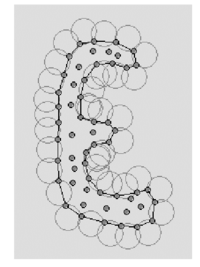Figure 2: Contour recognition: Empty circles with radius $\alpha h(x)$ define the boundary particles.

Once a decision has been made concerning which of the nodes are on the boundaries, the boundary surface must be defined. It is well known that in 3-D problems the surface fitting a number of nodes is not unique. For instance, four boundary nodes on the same sphere may define two different boundary surfaces, a concave one and convex one.

In this work, the boundary surface is defined with all the polyhedral surfaces having all their nodes on the boundary and belonging to just one polyhedron.

The correct boundary surface may be important to define the correct normal external to the surface. Furthermore; in weak forms (Galerkin) such as those used here a correct evaluation of the volume domain is also important. Nevertheless, it must be noted that in the criterion proposed above, the error in the boundary surface definition is proportional to ${\textstyle h}$. This is the error order accepted in a numerical method for a given node distribution. The only way to obtain more accurate boundary surface definition is by decreasing the distance between the nodes.

## 7 COMPUTATION OF THE CHARACTERISTIC LENGTHS

The evaluation of the stabilization parameters is one of the crucial issues in stabilized methods. Most of existing methods use expressions which are direct extensions of the values obtained for the simplest 1D case. In this work we will accept the so called SUPG assumption, i.e. to admit that vector ${\textstyle {\boldsymbol {h}}}$ has the direction of the velocity field [32-37]. This gives

 ${\boldsymbol {h}}=h_{s}{{\boldsymbol {u}} \over {u}}$
(43)

where ${\textstyle u=\vert {\boldsymbol {u}}\vert }$ and ${\textstyle h_{s}}$ as the “streamline” characteristic length

 $h_{s}=\max {\frac {({\boldsymbol {l}}_{j}^{T}{\boldsymbol {u}})}{u}}\quad ,\quad j=1,n_{s}$
(44)

where ${\textstyle {\boldsymbol {l}}_{j}}$ are the vectors defining the element sides (${\textstyle n_{s}=6}$ for tetrahedra).

A more consistent evaluation of ${\textstyle h}$ based on a diminishing residual technique can be found in .

## 8 EXAMPLES

All examples presented here have been obtained withthe fractional step algorithm described in Section 4.2.

### 8.1 Collapse of a water column

The first problem solved with the lagrangian formulation is the study of the collapse of a water column. This problem was solved by Koshizu and Oka  both experimentally and numerically. It has became a classical example to test the validation of the lagrangian formulation in fluid flows. The water is initially located on the left supported by a removable board. The collapse starts at time ${\textstyle t=0}$, when the removable board is removed. Viscosity and surface tension are neglected. Figure 3 shows the point positions at different time steps. The dark points represent the free-surface detected with the algorithms described in Section 5. The internal points are gray and the fixed points are black.

The water is running on the bottom wall until, near ${\textstyle 0.3}$ sec, it impinges on the right vertical wall. Breaking waves appear at ${\textstyle 0.6}$ sec. Around ${\textstyle 1}$ sec. the water reaches the left wall. Agreement with the experimental results of  both in the shape of the free surface as well as in the time evolution are excellent.

Figure 3 shows the point positions at different time steps. The darker points represent the free-surface detected.

The water is running on the bottom wall until, near ${\textstyle 0.3}$ sec, it impinges on the right vertical wall. Breaking waves appear at ${\textstyle 0.6}$ sec. Around ${\textstyle t=1}$ sec. the main water wave reaches the left wall again Agreement with the experimental results of ref.  both in the shape of the free surface as well as in the time development are excellent.

The 3D solution of the same problem is shown in Figure 4.

### 8.2 Semi-submerged rotating water mill

The second example is the analysis of a rotating water mill semi submerged in water. A schematic representation of a water mill is presented in Figure 5. The blades of the mill have an imposed rotating velocity, while the water is initially in a stationary and flat position. Fluid structure interactions with free-surfaces and water fragmentation are well reproduced in this example.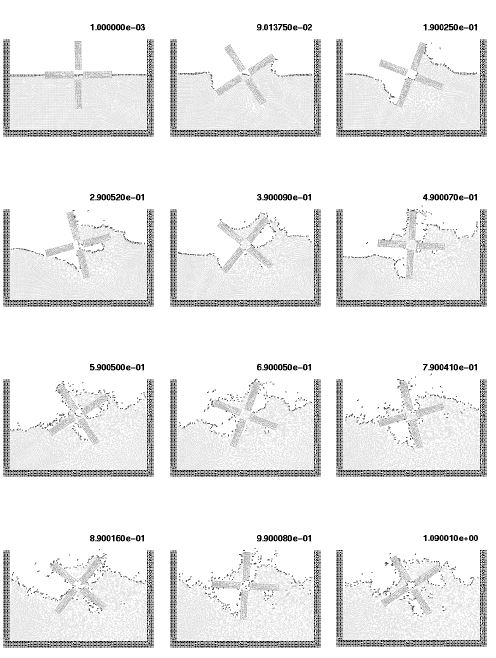Figure 5: Rotating water mill.

### 8.3 Sloshing problems

The simple problem of the free oscillation of an incompressible liquid in a container is considered next. Numerical solutions for this problem can be found in several references . This problem is interesting because there is an analytical solution for small amplitudes. Figure 6 shows a schematic of the problem, and represents also the point distribution in the initial position. The dark points represent the fixed points in which the velocity is fixed to zero.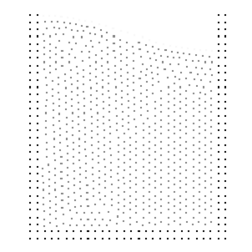Figure 6: Sloshing. Initial point distribution.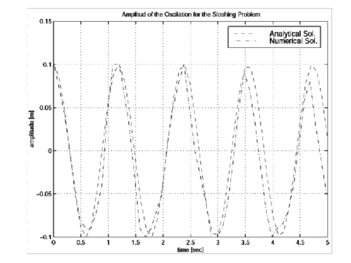Figure 7: Sloshing: Comparison of the numerical and analytical solution.

Figure 7 shows the variation in time of the amplitude compared with the analytical results for the near in viscid case. Little numerical viscosity is observed on the phase wave and amplitude in spite of the relative poor point distribution.

The analytical solution is only acceptable for small wave amplitudes. For larger amplitudes, additional waves are overlapping and finally, the wave breaks and also some particles can be separated from the fluid domain due to their large velocity. Figure 8 shows the numerical results obtained with the method presented in this paper for larger sloshing amplitudes. Breaking waves as well as separation effects can be seen on the free-surface. This particular and very complicated effect is apparently well represented by this model.Figure 8: Sloshing: Different time step for large amplitudes.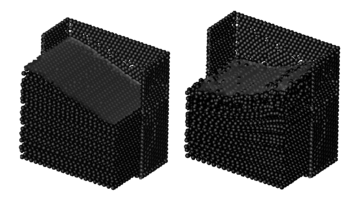Figure 9: Sloshing: Different time step for 3D domains.

In order to test the potentiality of the method in a 3D domain, the same sloshing problem was solved as a 3D problem. Figure 9 show the different point position at two time steps. Each point position was represented by a sphere and only a half of the fixed recipient is represented on the figure. This sphere representation is only used in order to improve the visualization of the numerical results.

### 8.4 Wave breaking on a beach

A simulation of the propagation of a water wave and its breaking due to shoaling over a plane slope is presented next. This example was numerically studied in  with a lagrangian formulation using directly the standard Finite Element Method with remeshing. There is also an analytical solution for a simplified approximation that is used for comparisons . Figure 10 shows the initial point distribution and Figure 11 a comparison with the analytical free-surface at different time step. The geometry of the problem as well as a discussion of the analytical solution may be found in .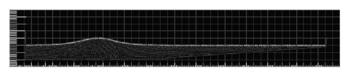Figure 10: Wave breaking on a beach. Initial geometry and point positions.

Initially (Figures 11.a. and 11.b.) the wave travels over a constant depth bottom towards the slope with no ostensible change of shape. Strongly non-linear effects appear when the wave hits the slope (Fig. 11.c.). The crest of the wave accelerates until it reaches the shore (Fig. 11.d.). At this time the comparisons with the analytical solution are in agreement only in the wave position. The shape of the wave obtained with the numerical solution is totally different. The reason is that the analytical solution gives symmetrical shape waves, which are not physical, before the breaking process. Subsequently, a water jet is formed at the crest plunge making the breaking wave (Figs. 11.e. and 11.f.) and coming in contact with the nearly still surface of the water ahead. In Ref. 44 the evaluation is stopped before this contact point. Using the methodology proposed in this paper, the analysis may be continued until the end. In Figs. 11.g. and 11.h. the wave finally hits a lateral wall (introduced in the model to stop the lateral effects) producing drop separations, and then coming back toward to the left as a new wave.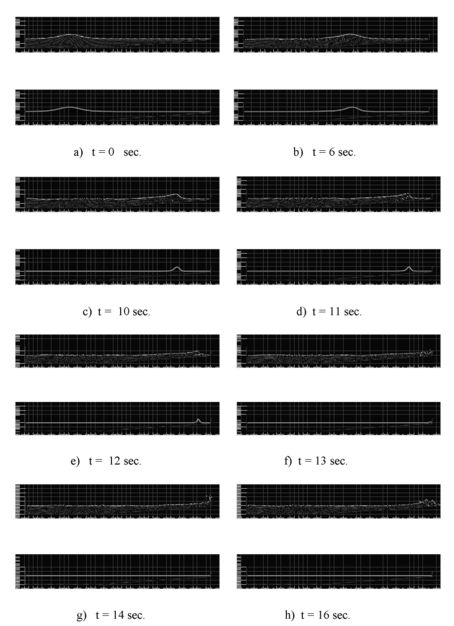Figure 11: Wave breaking on a beach. Comparison with analytical results at different time steps. Top: Numerical solution. Bottom: Analytical solution.

The ability of the model to accurately simulate the various stages of the wave breaking is noteworthy.

Nevertheless, a 2D domain is an easy case, and may be solved acceptably with any mesh generator. The true problems appears in a 3D domain, where the mesh generation is complicated with presence of slivers an other geometric mesh generation problems. In order to show the power of the tool presented, the same problem was solved in a 3D domain.

To transform the wave breaking described before in a true 3D problem, the initial position of the wave was introduced having an oblique angle with the beach line. In this way, a 3D effect appears. When the wave hits the slope, the crest of the wave accelerates differently accordingly with the depth, inducing the wave to correct its oblique position and break parallel to the beach. The results may be seen in Figure 12 for different time steps.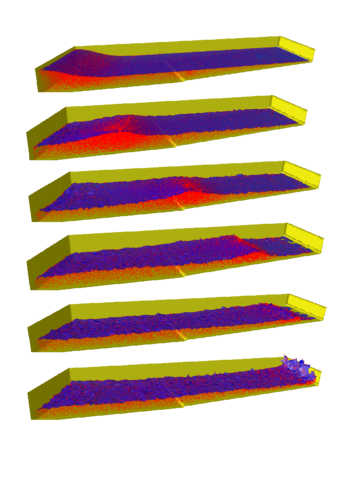Figure 12: Breaking wave on a beach: Oblique wave on a 3D domain.

### 8.5 Solid floating on a free-surface

The following example shown schematically in Figure 13 represents a very interesting problem of fluid structure interaction when there is a weak interaction between the fluid and a large rigid deformation of the structure. In this case, there is also a free-surface problem, representing a schematic case of see-keeping in ship hydrodynamics.Figure 13: Solid floating on a free-surface. Initial geometry and point distribution.

The example shows an initially stationary recipient with a floating piece of wood in which a wave is produced on the left side. The wave intercepts the wood piece producing a breaking wave and moving the floating wood. In this example the solid was represented by very viscous flows with a viscosity parameter order ten times greater than the water viscosity. Figure 14 shows the pressure contours and the free-surface position for different time steps.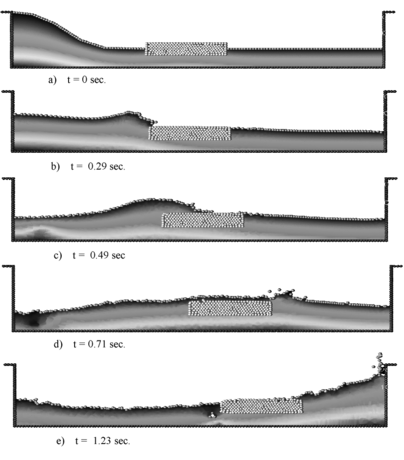Figure 14: Solid floating on a free-surface. Pressure contours and free-surface positions for different time step.

### 8.6 Solid cube falling in a recipient with water

This last example is also a case of fluid structure interaction. The solid is initially totally free and is falling down into a recipient with a fluid. In this example, the solid was modeled as a boundary condition for the fluid. Once the pressure and the viscous forces have been evaluated in the fluid, the solid is accelerated using Newton law. The solid has a mass and a gravity force concentrate in its gravity center. The solid is considered to be ligh compared to the liquid weight.

At the beginning the solid falls free due to the gravity forces (Figure 15). Once in contact with the water free-surface, the alpha-shape method recognizes the different boundary contours. The pressure and viscous forces are evaluated in all the domain and in particular on the solid contour. The flow forces introduce a negative acceleration in the vertical direction until , once the solid is completely inside the water, the vertical velocity becomes zero. Then, Arquimides forces bring the solid up to the free-surface. It is interesting to observe that there is a rotation of the solid. The reason is that the center of the floating forces is higher in the rotated position than in the initial ones.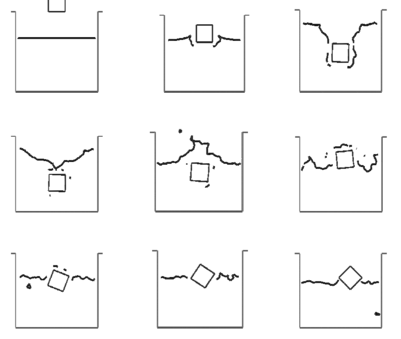Figure 15: Solid cube falling into a recipient with water. Free- surface position at different time steps.

## 9 CONCLUSIONS

The finite calculus expression of the fluid mechanics equations written in a lagrangian frame of reference is a good starting point for deriving stabilized finite element method for solving a variety of fluid flow problems. Both monolithic and fractional step algorithms with intrinsic stabilization properties can be readily derived as shown here. The lagrangian formulation allows to solve in an effective manner fluid flow problems involving large motions of the free surface and complex fluid-structure interactions.

## ACKNOWLEDGEMENTS

Thanks are also given to Mr. Romain Aubry for many useful discussions.

### Document informationPublished on 01/01/2003

### Document Score0

Views 15
Recommendations 0